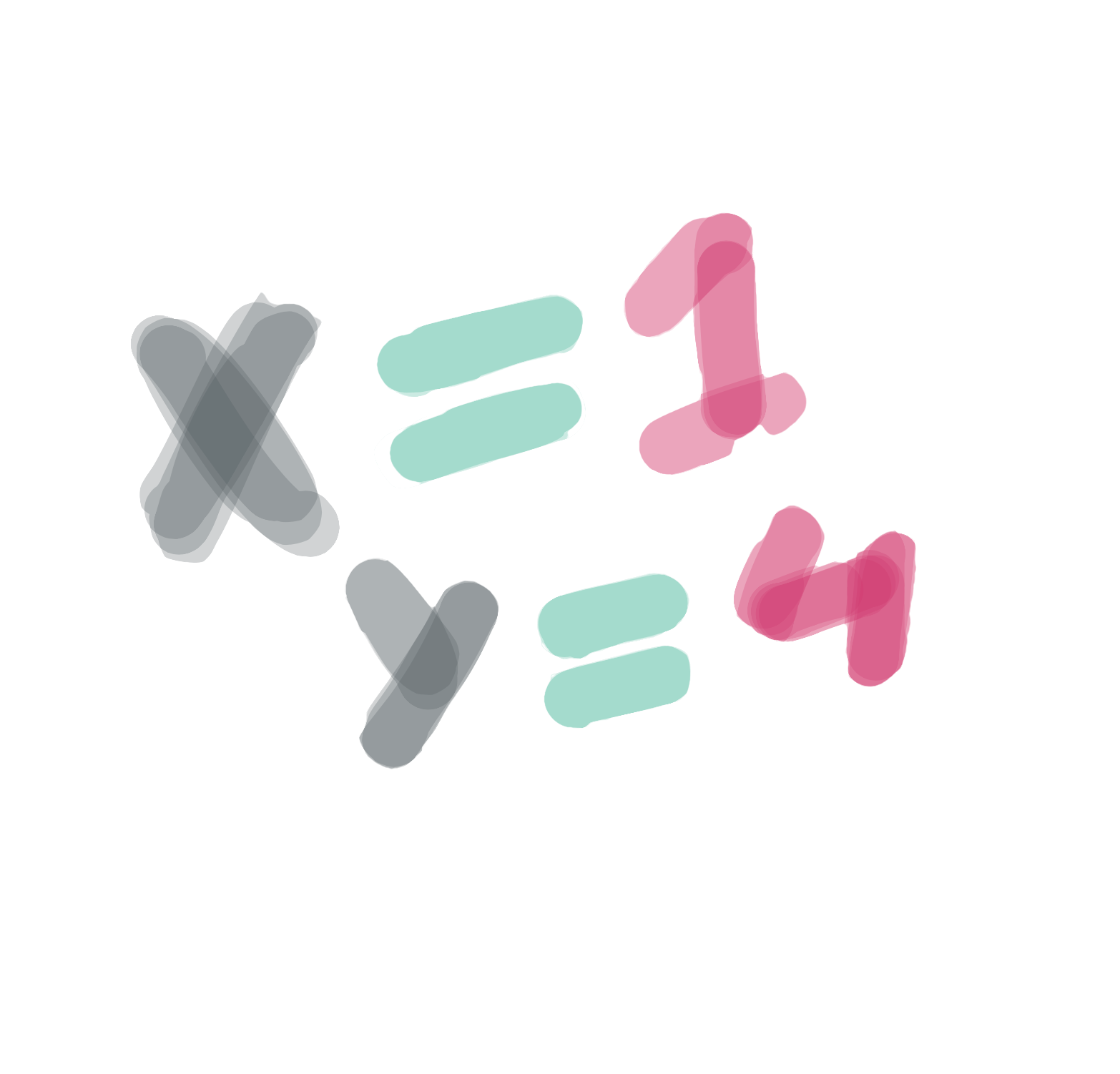# VariablesUntil now, we have always typed the numbers for our attributes directly, like this:

fill(255);

But in most programs, we don’t do this. Instead, we usually use something called a variable. When you write a variable, you can think of it as the computer remembering a number for you. When you want, you can ask the program the write the number back. Here’s how the same program looks with a variable:

int color = 255;
fill(color);

When we create a variable, we pick a name. We can give a variable any name we want, but it should only have letters - no numbers or spaces. Names are normally uncapitalized (e.g. `age` instead of `Age`), which might make your English teacher upset but makes programmers happy.

Not being able to use spaces is annoying, but there are a few ways we can deal with this. If we want to use a name with multiple words, such `My Favorite Color`, we either use Capital Letters to indicate the words: `myShoeSize`, or we use the underscore (`_`) letter: `my_shoe_size`. Either option works, so it comes down to which one you find prettier.

Here’s what some variables might look like:

int color = 255;
int myFavoriteColor = 255;
int age = 15;
int shoe_size = 8;

## Why do we need variables?

Variables are incredibly useful for a whole bunch of reasons, but for now we are going to focus on just one of them. Putting a number into a variable makes it really easy to change parts of your program quickly.

For example, let’s take a look at the face we drew before:

##### Code:
```ellipse(25,30,30,30);
ellipse(75,30,30,30);
rect(20,60,60,20);
```
##### Preview:

If we wanted to make the eyes bigger - say from size 30 to 50 - we would need to change every spot where we typed 30:

##### Code:
```ellipse(25,30,50,50);
ellipse(75,30,50,50);
rect(20,60,60,20);
```
##### Preview:

That’s a lot of typing! But if we create a variable to store our eye size, we only need to type the number once:

##### Code:
```int eyeSize = 50;
ellipse(25,30,eyeSize,eyeSize);
ellipse(75,30,eyeSize,eyeSize);
rect(20,60,60,20);
```
##### Preview:

Now that we’ve done it this way, we only need to change one number and both eyes will look different. Let’s try changing the size from 50 to 10:

##### Code:
```int eyeSize = 10;
ellipse(25,30,eyeSize,eyeSize);
ellipse(75,30,eyeSize,eyeSize);
rect(20,60,60,20);
```

## Calculating different numbers from our variables

We can also do math with our variables (eww, math!!) This means that we can write one number as a variable, and calculate a few numbers from it.

Let’s say we’re not sure how far our face should be from the left side of the screen. Rather than having to figure out where each eye and the mouth should be every time we want to make a change, we can create one variable called

int left = 20;

…and calculate the left side of each eye and the mouth from it. So, the left-most eye would change from..

ellipse(25,30,eyeSize,eyeSize);

… to …

ellipse(left + 5,30,eyeSize,eyeSize);

If we do the same thing for the other eye and the mouth, we get something that looks like this:

##### Code:
```int eyeSize = 20;
int left = 20;
ellipse(left + 5,30,eyeSize,eyeSize);
ellipse(left + 50,30,eyeSize,eyeSize);
rect(left,60,60,20);
```
##### Preview:

Now let’s say we want to change the size of the eyes and the location of the face. We can do this by just changing the value of the two variables

##### Code:
```int eyeSize = 50;
int left = 50;
ellipse(left + 5,30,eyeSize,eyeSize);
ellipse(left + 50,30,eyeSize,eyeSize);
rect(left,60,60,20);
```
##### Preview:
Exercise

Create a new variable called top, which determines where the top of the face will start. Update each eye and the mouth so their top attribute uses a calculation based on the top variable.

Next lesson Making the Screen Bigger